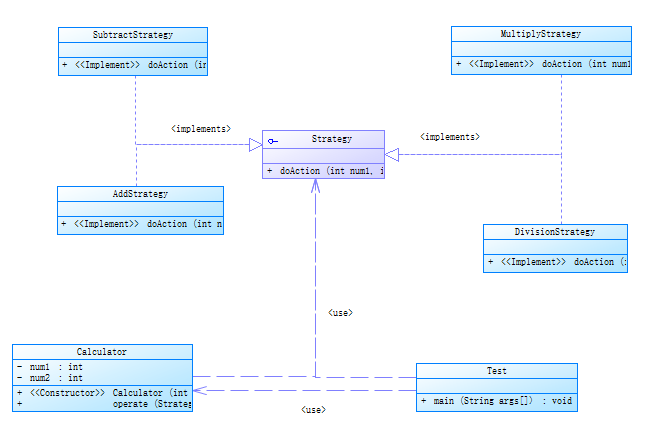java设计模式(二十一)--策略模式 顶原//策略接口
interface Strategy {
int doAction(int num1,int num2);
}

2.创建实现策略接口的四则计算实现类

public class AddStrategy implements Strategy {
@Override
public int doAction(int num1, int num2) {
return num1+num2;
}
}

public class SubtractStrategy implements Strategy{
@Override
public int doAction(int num1, int num2) {
return num1-num2;
}
}

public class MultiplyStrategy implements Strategy {
@Override
public int doAction(int num1, int num2) {
return num1*num2;
}
}

public class DivisionStrategy implements Strategy {
@Override
public int doAction(int num1, int num2) {
return num1/num2;
}
}

3.创建一个使用某种策略的类,可以根据需求不同来使用不同的策略

public class Calculator {
private int num1;
private int num2;
public Calculator(int num1, int num2) {
this.num1 = num1;
this.num2 = num2;
}
public int operate(Strategy strategy){
return strategy.doAction(num1, num2);
}
}

4.创建测试类,使用不同的策略来实现需求

public class Test {
public static void main(String[] args) {
System.out.println("=========加法计算器==========");
Calculator calculator=new Calculator(1, 2);
System.out.println("=========减法计算器==========");
Calculator calculator1=new Calculator(10, 20);
System.out.println("10-20="+calculator1.operate(new SubtractStrategy()));
System.out.println("=========乘法计算器==========");
Calculator calculator2=new Calculator(3, 5);
System.out.println("3*5="+calculator2.operate(new MultiplyStrategy()));
System.out.println("=========除法计算器==========");
Calculator calculator3=new Calculator(10, 2);
System.out.println("10/2="+calculator3.operate(new DivisionStrategy()));
}
}

5.后台输出

=========加法计算器==========

1+2=3
=========减法计算器==========

10-20=-10
=========乘法计算器==========

3*5=15
=========除法计算器==========

10/2=5

1.如果我们要加一个相反数策略的话,只需要实现策略接口即可,体现了易扩展性,符合”开闭原则”。
2.算法在客户端可以根据需要自由切换,适应了不同需求。
3.在客户端使用将策略对象参数化的方法来调用具体策略的方法,避免在客户端新建对象实例,提高系统性能。

1.策略使用类要知道所有的策略类才行,然后才能自行决定要用哪一个策略。
2.随着策略的不断增多,策略类也会不断的增多,提高后期的维护困难性。

book: 阎宏《JAVA与模式》 架构设计栏目 http://blog.csdn.net/enterprise/column.html 概要： http://bbs.csdn.net/forums/Embeddeddriver 23种设计模式分别是： 1.单例模式　2.工厂方法模式...

jayronwang
2014/12/19
296
0

Template Method模板方法设计模式定义一个操作中算法的骨架，将具体步骤的执行延迟到子类中实现。Java中的抽象类就是使用了模板方法设计模式。模板方法设计模式结构如下： 以文档处理为例，T...

2014/01/24
221
0

2018/05/08
281
0

2018/09/03
0
0

GitChat技术杂谈
2018/10/26
0
0

Netty整合Protobuffer

18分钟前
10
0

BWH_Steven
37分钟前
3
0

1编写一个程序，提示用户输入名和姓，然后以“名,姓”的格式打印出来。 #include <stdio.h>#include <stdlib.h> int main(){ char firstName; char lastName; print......

1李嘉焘1
49分钟前
8
0

76
0
Docker 可视化管理 portainer

Moks角木

9
0